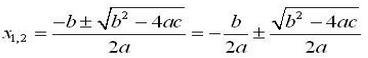# 2020年哈尔滨工业大学C语言程序设计精髓 第四周练兵编程题

/本文为原创内容，C语言练习试题适用参考学习，因为全为自己编写可能没有按照标准答案或者含有使可读性减少的步骤，有不对的地方希望各位指出/

1

123a↙

Input error!

%d\n” （注意：等号的两边各有一个空格）

#include<stdio.h>
int main()
{
int a,b,c;
c=scanf("%d %d",&a,&b);
if(c==2)
{
printf("a = %d, b = %d\n",a,b);
}
else
{
printf("Input error!");//选择分支
}
return 0;
}

2

（1）能被4整除，但不能被100整除；

（2）能被400整除。

2015↙

No

2016↙

Yes

-123↙

Input error!

a↙

Input error!

#include <stdio.h>
#define EPS 1e-6
int main()
{
int year,num;
num=scanf("%d",&year);
if(num==1&&year>EPS)//保证数据偏小接近0也能被筛选
{
if(year%100!=0&&year%4==0||year%400==0)
{
printf("Yes\n");
}
else
{
printf("No\n");
}
}
else
{
printf("Input error!\n");
}
return 0;
}

3

#include<stdio.h>
int main()
{
int score;
char grade;
printf("Please input
score:");
scanf("%d", &score);
if (score < 0 || score > 100)
printf("Input error!\n");
else if (score >= 90)
grade = 'A’;
else if (score >= 80)
grade = 'B';
else if (score >= 70)
grade = 'C';
else if (score >= 60)
grade = 'D';
else
grade = 'E';
printf("grade:%c\n", grade);
return 0;
}

Please input score:

-1↙

Input error!

Please input score:

95↙

grade: A

Please input score:

82↙

grade: B

Please input score:

72↙

grade: C

Please input score:

66↙

grade: D

Please input score:

32↙

grade: E

Please input score:

127↙

Input error!

#include<stdio.h>
#define EPS 1e-6
int main()
{
int score,num;
char grade;
printf("Please input score:\n");
num=scanf("%d", &score);
if(num==1&&score>=EPS&&score<=100)//筛选数据排除0~100以外的数据
{
if (score >= 90)
grade = 'A';
else if (score >= 80&&score<90)
grade = 'B';
else if (score >= 70&&score<80)
grade = 'C';
else if (score >= 60&&score<70)
grade = 'D';
else
grade = 'E';
printf("grade: %c\n", grade);
}
else
{
printf("Input error!\n");
}
return 0;
}

4

is other character.

Input simple:

b↙

It is an English character.

Input simple:

6↙

It is a digit character.

Input simple:

*↙

It is other character.

Input simple:

A↙

It is an English character.

“%c”

character.\n”

character.\n”

character.\n”

#include<stdio.h>
int main()
{
char n;
printf("Input simple:\n");
scanf("%c",&n);
if (n>='a'&& n<='z'|| n>='A' && n<='Z')//判断字母
{
printf("It is an English character.\n");
}
else if(n>='0'&&n<='9')//判断数字
{
printf("It is a digit character.\n");
}
else//不满足以上条件其他字符
{
printf("It is other character.\n");
}

return 0;
}

5

0区

1区

2区

3区

4区

1500公里（含）以内

1500——2500公里

2500公里以上

（1） 起重（首重）1公斤按起重资费计算（不足1公斤，按1公斤计算），超过首重的重量，按公斤（不足1公斤，按1公斤计算）收取续重费；

（2） 同城起重资费10元，续重3元/公斤；

（3） 寄往1区（江浙两省）的邮件，起重资费10元，续重4元；

（4） 寄往其他地区的邮件，起重资费统一为15元。而续重部分，不同区域价格不同：2区的续重5元/公斤，3区的续重6.5元/公斤，4区的续重10元/公斤。

4,4.5↙

Price: 55.00

5,3.2↙

Error in Area

Price:
0.00

#include<stdio.h>
int main()
{
int n;
float Price,w;
scanf("%d,%f",&n,&w);
if(n>=0&&n<=4)
{
if(n==0)
{
if(w<=1)
{
Price=10;
}
else
{
if(w-(int)(w)==0)
Price=(w-1)*3.0+10;
else
Price=(int)(w)*3.0+10;
}
}
if(n==1)
{
if(w<=1)
{
Price=10;
}
else
{
if(w-(int)(w)==0)
Price=(w-1)*4.0+10;
else
Price=(int)(w)*4.0+10;
}
}
if(n==2)
{
if(w<=1)
{
Price=15;
}
else
{
if(w-(int)(w)==0)
Price=(w-1)*5.0+15;
else
Price=(int)(w)*5.0+15;
}
}
if(n==3)
{
if(w<=1)
{
Price=15;
}
else
{
if(w-(int)(w)==0)
Price=(w-1)*6.5+15;
else
Price=(int)(w)*6.5+15;
}
}
if(n==4)
{
if(w<=1)
{
Price=15;
}
else
{
if(w-(int)(w)==0)
Price=(w-1)*10.0+15;
else
Price=(int)(w)*10.+15;
}
}

printf("Price: %5.2f\n",Price);
}
else
{
printf("Error in Area\n");
printf("Price: %5.2f\n",Price);
}

return 0;
}

6

Please input n:

1200↙

12,0

sum=12,sub=12,multi=0

The second operator is zero!

Please input n:

-2304↙

-23,-4

sum=-27,sub=-19,multi=92

dev=5.75,mod=-3

second operator is zero!\n”

#include<stdio.h>
#include<math.h>
int main()
{
int num,a,b;
printf("Please input n:\n");
scanf("%d",&num);
if(num<0)//判断正负，进行不同的分位
{
a=-(fabs(num)/100);//千位百位
b=-(fabs(num)+a*100);//十位个位
printf("%d,%d\n",a,b);
}
else
{
a=fabs(num)/100;
b=fabs(num)-a*100;//如上
printf("%d,%d\n",a,b);
}
if(b==0)
{
printf("sum=%d,sub=%d,multi=%d\n",a+b,a-b,a*b);
printf("The second operator is zero!\n");
}
else
{
printf("sum=%d,sub=%d,multi=%d\n",a+b,a-b,a*b);
printf("dev=%.2f,mod=%d\n",(float)(a)/b,a%b);
}
return 0;
}

7

Input distance and time:2,2↙

fee = 8

Input distance and time:5,5↙

fee = 14

Input distance and time:12,15↙

fee = 34

Input distance and time:20,0↙

fee = 52

#include<stdio.h>
#include<math.h>
int main()
{
float distance;
int time;
printf("Input distance and time:");
scanf("%f,%d",&distance,&time);
if(distance<=3)
{
if(time<=4)
{
printf("fee = %.0f\n",8.0);
}
else
{
printf("fee = %.0f\n",8.0+(time/5)*2);
}
}
else if(distance<=10)
{
if(time<=4)
{
printf("fee = %.0f\n",(distance-3)*2+8);
}
else
{
printf("fee = %.0f\n",(distance-3)*2+8+(time/5)*2);
}
}
else
{
if(time<=4)
{
printf("fee = %.0f\n",(distance-10)*3+22);
}
else
{
printf("fee = %.0f\n",(distance-10)*3+22+(time/5)*2);
}
}
return 0;
}

8

Please enter the number:

2563↙

2563: 1000-9999

Please enter the number:

156↙

156: 100-999

Please enter the number:

36↙

36: 10-99

Please enter the number:

3↙

3: 0-9

Please enter the number:

10923↙

error!

number:\n”

“%d: 1000-9999\n”

“%d: 100-999\n”

“%d: 10-99\n”

“%d: 0-9\n”

#include<stdio.h>
int main()
{
int n;
printf("Please enter the number:\n");
scanf("%d",&n);
if(n>=1&&n<=9)//注意区间
printf("%d: 0-9\n",n);
else if(n>=10&&n<=99)
printf("%d: 10-99\n",n);
else if(n>=100&&n<=999)
printf("%d: 100-999\n",n);
else if(n>=1000&&n<=9999)
printf("%d: 1000-9999\n",n);
else
printf("error!\n");
return 0;
}

9Please enter the coefficients a,b,c:

1,2,1↙

x1=-1.0000, x2=-1.0000

Please enter the coefficients a,b,c:

2,6,1↙

x1=-0.1771, x2=-2.8229

Please enter the coefficients a,b,c:

2,1,6↙

error!

a,b,c:\n”

#include<stdio.h>
#include<math.h>
int main()
{
float a,b,c,x,y;
printf("Please enter the coefficients a,b,c:\n");
scanf("%f,%f,%f",&a,&b,&c);
x=-b/(2*a);
y=b*b-4*a*c;
if(y<0)//判断dengta的正负
{
printf("error!\n");
}
else
{
printf("x1=%7.4f, x2=%7.4f\n",x+sqrt(y)/(2*a),x-sqrt(y)/(2*a));//直接带公式
}
return 0;
}03-18117
02-243292
04-16906
05-071万+
01-231万+
01-232万+
12-051087
04-193360
02-107997
11-118905
11-211290
©️2020 CSDN 皮肤主题: 游动-白 设计师:白松林